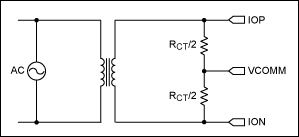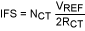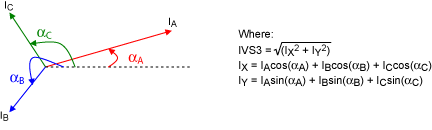# How to Conduct Three-Current Vector Sum Measurements

### Abstract

Tampering with electric energy measurement causes significant revenue loss to the energy providers. The MAXQ3183 polyphase AFE provides current vector sum measurements for tamper detection. This application note describes how to configure the MAXQ3183 for three-current vector sum. Test results generated with a reference design meter are provided.

### Introduction

Tampering with the measurement of electric energy occurs in a variety of forms and causes significant revenue loss to the energy providers. By some estimates¹ as much as 40% of revenue has been lost to energy theft in some markets. A common method of tampering is to bypass the measurement sensors outside the energy meter, but there is a way to check for this. By monitoring the imbalance between the neutral current and the three-phase currents in a three-phase energy meter, you can detect current bypass connections.

In a normal three-phase system the instant neutral current measured in real time is the vector sum of the three phase currents, because the neutral current is the only return path for all the phase currents. In the event of any attempted current bypass, the balance among the currents is broken. See Figure 1. Therefore, one can monitor the current balance, look for an imbalance at every data sample, and then issue an alert when an imbalance is found. Alternatively, the energy provider can decide only to start the monitoring process after enough reliable evidence of "normal" operation has been collected. Alerting at every sample may cause too many interrupts to the system, and is often not necessary for most systems.

Comparing the RMS value of the neutral current with the vector sum of the three phase currents is a natural choice. The accumulation process in the RMS calculation filters out spikes in data samples due to noise and, therefore, eliminates false alarms. An even more convenient way is to monitor the vector sum of the neutral current together with the three phase currents, and then interrupt the host only if the RMS of this four-current sum exceeds a preset threshold. This application note discusses current bypass detection using the current vector sum features of the MAXQ3183, a low-power multifunction polyphase AFE.Figure 1. Current bypass on phase B.

### Vector Sum Measurement Modes

The MAXQ3183 provides two modes of current vector sum measurement: IVS3 and IVS4. The IVS3 mode sums the three phase currents, and the IVS4 mode sums the three phase currents plus the neutral current.

To use ISV3 for bypass detection, the host controller periodically commands the MAXQ3183 to do an ISV3 measurement (returned in RMS) and to compare it against the neutral current (RMS, also measured on demand). If the difference is higher than a threshold value, then a bypass event is detected.

A more convenient approach uses the ISV4. In this method a threshold is set in the ISUMLVL register and the corresponding interrupt bit (IRQ_MASK.EISUM) enabled. The MAXQ3183 would generate an interrupt when the RMS of the four-current vector sum exceeds the threshold. Using the interrupt feature the host is contacted only when necessary, instead of the host constantly polling the vector sum register to determine if an over-the-limit event has occurred. Selection of the vector sum measurement-mode is configured in the AUX_CFG register.

The following registers will be configured and accessed during a vector sum measurement.

 Register Address Size (bits) Short Description AUX_CFG 0x010 16 Auxiliary channel configuration SCAN_IN 0x00E 8 Time slot assignment: neutral current channel I.HARM 0x840 64 A virtual register for neutral current RMS/harmonic component/vector sum (IVS3 or IVS4) A.IRMS 0x1CC 32 Current RMS, phase A B.IRMS 0x2B8 32 Current RMS, phase B C.IRMS 0x3A4 32 Current RMS, phase C N.IRMS 0x11C 16 Neutral current RMS/harmonic component/vector sum (IVS3 or IVS4)

Description of the SCAN_IN register.

 Bit Name Function 7:4 ADCMX Analog Conversion Select. This four-bit field determines which of the following analog inputs are sampled during this time slot. All other values are reserved. By default, this register is set to 0110. 0000 = V0P - VN 0001 = V1P - VN 0010 = V2P - VN 0011 = I0P - I0N 0100 = I1P - I1N 0101 = I2P - I2N 0110 = INP - VN 1xxx = Temperature 3 DADCNV ADC Disable. When set, disables the ADC for this time slot. 2:0 — Reserved.

Description of the AUX_CFG register.

 Bit Name Function 15:13, 4 — Reserved. 12:8 ORDH Order of Harmonic (1–21). The output of harmonic voltage is read via virtual register 0x830 and current at 0x840. 7 ENHARM Enable Auxiliary Channel Harmonic Filter. When set, the auxiliary channel is processed through the harmonic filter. The parameters for this filter can be set in the B0HARM and A1HARM registers. 6 ENAUX Enable Auxiliary Channel. When set, enables auxiliary channel processing. 5 INREV Sets the sign of the neutral current, used in the vector sum calculation.0 = positive, i.e., IN is directly summed with IA + IB + IC.1 = negative, -IN is summed with IA + IB + IC. 3:0 AUX_MUX Auxiliary Channel Input or Current Vector Sum Select. The lower three bits select the input to be processed by the auxiliary channel if the MSB (bit 3) is cleared. When MSB is set, this field selects vector sum computation: 1001 = IA + IB +IC 1111 = IA + IB + IC + IN (INREV = 0) or IA + IB + IC - IN (INREV = -1) 0001 = IN 0010 = VA 0011 = IA 0100 = VB 0101 = IB 0110 = VC 0111 = IC All other values are reserved.

### Configuring for IVS4

To use the IVS4 mode, neutral current monitoring must be enabled (by default, the neutral-current channel monitoring is off). There are two registers that govern the neutral-current monitoring: AUX_CFG and SCAN_IN. Set the AUX_CFG.ENAUX bit to enable the "processing of the auxiliary channel," and clear the SCAN_IN.DADCNV bit to enable the ADC conversion on the neutral current channel. This two-step procedure is required because AUX_CFG specifies what the DSP processor should compute in the time slot allocated to the auxiliary channel, which is independent of what is happening with the neutral channel's ADC operation. The auxiliary channel processing can be configured as one of the following:

1. Compute the RMS of neutral current.
2. Compute the harmonic component of any of the phase voltages or currents.
3. Compute IVS3 or IVS4.
In the IVS4 mode, neutral-current channel is sampled for computing the IVS4 only; RMS of the neutral current is not available. The procedure for configuring ISV4 measurement is outlined below:
1. Enable ADC conversion on the neutral current channel by clearing the SCAN_IN.DADCNV bit and setting the SCAN_IN.ADCMX bits (7:4) to 0110. SCAN_IN should contain 0x060.

2. Enable auxiliary processing for the four-current vector sum computation by setting the AUX_CFG.ENAUX bit and setting the AUX_CFG.AUX_MUX bits (3:0) to 1111. Clear all other bits. The AUX_CFG register should contain 0x004F.

3. Wait for 3to 4 seconds for filters to settle and the computation to complete, then read vector sum from N.IRMS (0x11C) or I.HARM (0x840). If reading from I.HARM, make sure that the AMP_CC register is set properly.
Note that the four-current vector sum also supports summing with the reverse neutral current: IA + IB + IC - IN. This feature is useful if one finds that the neutral current-sensor connection is reversed. To do this, set the INREV bit in the AUX_CFG register so that AUX_CFG=0x006F.

### Configuring for IVS3

The IVS3 mode does not use neutral current measurement, so the ADC conversion is disabled (the default condition).

1. Enable auxiliary processing for three-current vector sum computation by setting the AUX_CFG.ENAUX bit and setting the AUX_CFG.AUX_MUX bits (3:0) to 1001. Make sure that all other bits are cleared. The AUX_CFG register should contain x0049.

2. Wait for 3 to 4 seconds for filters to settle and computation to complete, then read vector sum from N.IRMS (0x11C) or I.HARM (0x840). If reading from I.HARM, make sure that the AMP_CC register is set properly.

### Test Setup

The test setup is illustrated in Figure 2.

Meter AC specifications:
UN = 220V
IMAX = 9A
Frequency = 50Hz/60Hz

Current transformer specifications:
1.5(9)mA/5mA
Current = 9A (max)
Burden resistor: 20Ω
Linearity error: 0.05%

Meter tester: MTE PCS400.3

Note: this tester does not support neutral current testing. It can only source three currents. This application note discusses testing three-current vector sum (ISV3). Results for four-current vector sum tests will be discussed in a follow-up application note.

### Determining the Conversion Parameters

The MAXQ3183 processes and presents numbers in integers only. To present the measurement results to the energy providers and consumers, those integers need to be converted to the appropriate physical units. Two design parameters are needed for converting the MAXQ3183 register values in meter units to physical units: VFS and IFS. VFS is the AC-input voltage that would produce a voltage amplitude of VREF/2 on the MAXQ3183's voltage pins, where VREF is the MAXQ3183's internal reference voltage level and is typically 2.048V. IFS is the AC-input current that would produce a voltage amplitude of VREF/2 on the MAXQ3183's current pins.

For the MAXQ3183 reference design, the voltage sensing circuit consists of eight 68kΩ resistors and a 1kΩ resistor-divider (Figure 3). (For more information about the reference design, see application note 4663, "Reference design for an energy-meter AFE using the MAXQ3180/MAXQ3183."Figure 3. Voltage sensing circuit on the MAXQ3183.

We calculate VFS as:Where:
R1 = eight 68kΩ
R2 = 1kΩ
VREF = 2.048V
VFS = (eight × 68 + 1) × 2.048/2 ~ 558V

Therefore, VFS = 558V.

The current-sensing circuit of the reference design consists of a current transformer with a primary/secondary turn ratio = 300, and a burden resistor of 20Ω. The CT secondary output is center biased at VCOMM (Figure 4).Figure 4. Current sensing circuit on the MAXQ3183.

We calculate IFS as:Where:
NCT = 300
RCT = 20Ω
VREF = 2.048V
IFS = 300 × 2.048/(2 × 20) ~ 15A

Therefore, IFS = 15A.

Vector sum computation sums the real-time ADC samples of three (or four) current vectors. The accuracy of the computation is affected by errors in both the magnitudes and phase angles of the current vectors. Therefore, it is important that gains and phase angles be properly calibrated before performing vector sum measurement. See the MAXQ3183 data sheet and related application notes for details on meter calibrations.

### Single-Phase Current (IA)

This test scenario is very simple, but yet interesting because it illustrates the various error contributions in the measurement. See the earlier section Configuring for IVS3 for instructions on configuring the MAXQ3183 for three-current vector sum measurement. Set IB = IC = 0 on the meter tester. Vary IA from 10A to 0.02A, and collect the A.IRMS and IVS3 (from N.IRMS).

 Source, IA (A) = 10 6 1 0.1 0.05 0.02 A.IRMS (1CC) 00AAB58E 00667D99 00111348 0001B3B2 0000DA44 0000589F B.IRMS (2B8) 0000089A 000008A4 00000293 0000028F 000002BD 000002CB C.IRMS (3A4) 00000222 0000025B 0000022E 00000276 00000280 000002A8 N.IRMS (11C) 00AAB397 00667405 00111319 0001B66A 0000DF35 00006586 IRMS in (A) A.IRMS 10.002 6.005 1.001 0.100 0.050 0.020 B.IRMS 0.002 0.002 0.001 0.001 0.001 0.001 C.IRMS 0.000 0.001 0.000 0.001 0.001 0.001 N.IRMS 10.002 6.003 1.000 0.100 0.051 0.023 IA Err (%) 0.02% 0.09% 0.05% -0.28% -0.09% 1.42% IVS3 Exp (A) 10.00 6.00 1.00 0.10 0.05 0.02 IVS3 Err (%) 0.02% 0.05% 0.05% 0.35% 2.18% 16.18%

Note that B.IRMS and C.IRMS both show nonzero values. These residual values derive from noises in the system, since the source supplies are set to IB = IC = 0. The same noises contribute to the IVS3 errors, which become significant at low inputs (I < 0.1A). Note that the error of ISV3 is much higher than that of the IA at IA = 0.05A and 0.02A. This is mainly because noises from phase B and phase C still contribute to the IVS3 measurement, while they do not contribute to the IA measurement.

### Three-Phase Currents (IA + IB + IC)

For balanced three-phase loads, the three-vector sum should be zero. This test result may serve as the reference for setting the threshold level to be used with the ISV4. Vary IA (= IB = IC) from 10A to 0.05A and collect A.IRMS, B.IRMS, C.IRMS, and IVS3 (from N.IRMS).

 Source 10 6 1 0.1 0.05 A.IRMS (1CC) 00AAAE2A 0066619F 001112C4 0001B610 0000DABC B.IRMS (2B8) 00AAAB48 00666352 0011111B 0001B665 0000DC3A C.IRMS (3A4) 00AAB08A 0066665C 00111149 0001B3E3 0000DA16 N.IRMS (11C) 00008B7E 00007652 00006D17 000033F2 00003729 X.IRMS in A A.IRMS 10.001 5.999 1.000 0.100 0.050 B.IRMS 10.000 5.999 1.000 0.100 0.050 C.IRMS 10.001 6.000 1.000 0.100 0.050 IVS3 in A N.IRMS 0.032 0.027 0.025 0.012 0.013 IVS3 Exp (A) 0.00 0.00 0.00 0.00 0.00 IVS3 Err (A) 0.032 0.027 0.025 0.012 0.013

The above result indicates that for the meter under test, the vector sum measurement error is as high as 0.03A. Setting a threshold at or below this level would certainly generate false alarms.

### Three-Phase Currents (IA + IB + IC), Unbalanced Loads

Set IB = IA/2, IC = IA/4 on the meter tester; vary IA from 10A to 0.05A and collect the A.IRMS, B.IRMS, C.IRMS, and IVS3 (from N.IRMS).

 Source, IA = 10 6 1 0.1 0.05 A.IRMS (1CC) 00AAACF5 0066652C 0011114E 0001B43E 0000DAEA B.IRMS (2B8) 00555225 0033331F 00088E13 0000DD04 00006E38 C.IRMS (3A4) 002AAEE9 0019A09B 00044478 00006C38 00003459 N.IRMS (11C) 0070F034 0043BC89 000B4ACA 00012574 00009AD6 X.IRMS in (A) A.IRMS 10.000 6.000 1.000 0.100 0.050 B.IRMS 4.999 3.000 0.501 0.051 0.025 C.IRMS 2.501 1.502 0.250 0.025 0.012 IVS3 in A N.IRMS 6.617 3.969 0.662 0.067 0.035 IVS3 Exp (A) 6.614 3.969 0.661 0.066 0.033 IVS3 Err (%) 0.1% 0.0% 0.1% 1.8% 7.4%

The expected IVS3 values are computed from the following equation:### Vector Sum Error Sources: a Theoretical View

A simple spreadsheet calculation below illustrates that the performance of vector sum calculation is sensitive to errors in both the phase angles and the magnitudes of the current vectors.In the table below, the ISV3 Err column is the relative error of ISV3 with respect to the base case (row 1). Phase-angle errors are introduced in phase B phase angle αB. The table shows that a 0.5° phase-angle error contributes about 1% error in the vector sum measurement under the input conditions evaluated.

 IRMS IA3 IB4 IC1 ISV3 (A) ISV3 Err (%) Angle 0.0 240.0 120.0 2.646 Angle 0.0 240.5 120.0 2.669 0.86% Angle 0.0 241.0 120.0 2.692 1.73% Angle 0.0 242.0 120.0 2.737 3.46%

The next table shows that an error in the magnitude (RMS) of phase current contributes approximately the same amount of relative error to the vector sum measurement.

 Angle IA0 IB240 IC120 ISV3 (A) ISV3 Err (%) IRMS 3.0 4.00 1.0 2.646 IRMS 3.0 4.02 1.0 2.661 0.57% IRMS 3.0 4.04 1.0 2.676 1.15% IRMS 3.0 4.08 1.0 2.707 2.30%

### Conclusions

The MAXQ3183 computes three-current vector sum and four-current vector sum, both as on-demand features. Users must configure multiple registers to command a vector-sum measurement. This application note illustrated the configuration procedure with multiple examples of three-current vector sum measurements. Test results were provided as reference cases for customer design validations. The results illustrate and underscore the accuracy of the vector-sum measurement which is, however, sensitive to errors in both the magnitudes and phase angles of the current vectors. Designers should pay close attention to the calibration of both the gain factors and phase angles.

¹Indian Officials Wage War on Energy Theft (http://dailyreporter.com/blog/2009/07/06/indian-officials-wage-war-on-energy-theft/); India Struggles with Power Theft (http://news.bbc.co.uk/2/hi/business/4802248.stm).